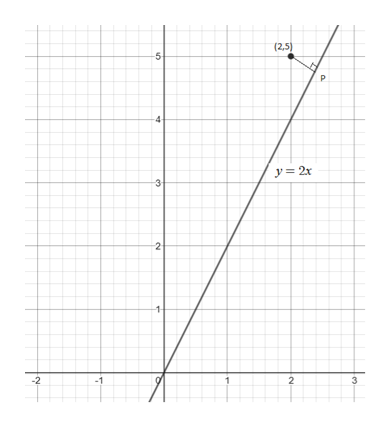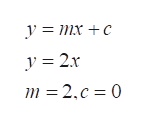find the distance from the point (2,5) to the line y=2x.

Question

find the distance from the point (2,5) to the line y=2x.

Step 1

Refer  to the question,

The distance from a point to the line is the shortest distance between the point or ay point on the line.

So the graph of the above equation and point is,help_outlineImage Transcriptionclose(2,5) 5 P 4 y2x 3 2 1 3 -2 2 en fullscreen
Step 2

Find the point p on the lne y=2x, as the slope of the line is 2 when compared with the slope intercept form y=mx +c , where m is the slope and c is the  intercept.help_outlineImage Transcriptionclosey = mx +c y = 2r m = 2.c 0 fullscreen
Step 3

As the perpendicular is draw from the given point on the line y=2x so for two lines ...

Want to see the full answer?

See Solution

Want to see this answer and more?

Our solutions are written by experts, many with advanced degrees, and available 24/7

See Solution
Tagged in

Other